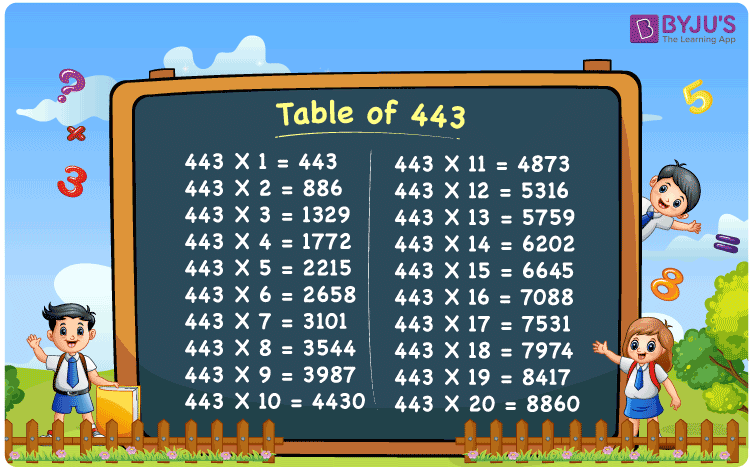Checkout JEE MAINS 2022 Question Paper Analysis : Checkout JEE MAINS 2022 Question Paper Analysis :

# Table of 443

The table of 443 describes the values of the product of the actual number with consecutive natural numbers. The multiplication of 443 with any other number expresses the repeated addition of 443, according to the number to which it is multiplied. For example, 443 x 4 = 443 + 443 + 443 + 443 = 1772. Learn more multiplication tables here at BYJU’S. Students can also download the PDF of the 443 times table here for free.

## Table of 443 Chart## What is the 443 Times Table?

The multiplication table of 443 is the continuous addition of 443. The table of 443 expresses the values we get when 443 is multiplied by another natural number. From the table given below, learn the table of 443 up to 10 times for quick and easy calculations of problems.

 Using multiplication Using repeated addition 443 × 1 = 443 443 443 × 2 = 886 443 + 443 = 886 443 × 3 = 1329 443 + 443 + 443 = 1329 443 × 4 = 1772 443 + 443 + 443 + 443 = 1772 443 × 5 = 2215 443 + 443 + 443 + 443 + 443 = 2215 443 × 6 = 2658 443 + 443 + 443 + 443 + 443 + 443 = 2658 443 × 7 = 3101 443 + 443 + 443 + 443 + 443 + 443 + 443 = 3101 443 × 8 = 3544 443 + 443 + 443 + 443 + 443 + 443 + 443 + 443 = 3544 443 × 9 = 3987 443 + 443 + 443 + 443 + 443 + 443 + 443 + 443 + 443 = 3987 443 × 10 = 4430 443 + 443 + 443 + 443 + 443 + 443 + 443 + 443 + 443 + 443 = 4430

## Multiplication Table of 443

The multiplication table of 443 is given below up to 20 times and these values are called the first 20 multiples of 443.

 443 × 1 = 443 443 × 2 = 886 443 × 3 = 1329 443 × 4 = 1772 443 × 5 = 2215 443 × 6 = 2658 443 × 7 = 3101 443 × 8 = 3544 443 × 9 = 3987 443 × 10 = 4430 443 × 11 = 4873 443 × 12 = 5316 443 × 13 = 5759 443 × 14 = 6202 443 × 15 = 6645 443 × 16 = 7088 443 × 17 = 7531 443 × 18 = 7974 443 × 19 = 8417 443 × 20 = 8860

## Example on the Table of 443

Question:

Using the table of 443, find the value of 443 times 10 plus 443 times 14.

Solution:

Using the table of 443, 443 × 10 = 4430 and 443 × 14 = 6202.

Now,

443 times 10 plus 443 times 14 = 443 × 10 + 443 × 14

= 4430 + 6202

= 10632

Therefore, the value of 443 times 10 plus 443 times 14 is 10632.

This value is also equivalent to 443 times 24.

## Frequently Asked Questions on Table of 443

### What is the multiplication table of 443?

The multiplication table for 443 is 443 × 1 = 443, 443 × 2 = 886, 443 × 3 = 1329, 443 × 4 = 1772, 443 × 5 = 2215, and so on.

### How many times of 443 is 3987?

Nine times of 443 or 443 times 9 is 3987. That means, by multiplying 443 by 9 we get 3987, i.e. 443 × 9 = 3987.

### How do you write the value of 443 times 7 using addition?

We can write the value of 443 times 7 using the repeated addition method as shown below.
443 + 443 + 443 + 443 + 443 + 443 + 443 = 3101